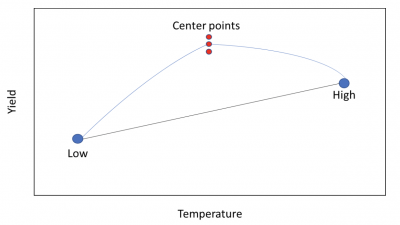Center points are used in the context of doing Design of Experiments (DOE). These are additional runs in the mathematical center of two continuous variables. Let’s explore this a little further to see the value of using center points when you set up your DOE.

## Overview: What are center points?

A design of experiments (DOE) is the perfect tool for understanding the relationship of your input and output process variables. In reality, DOE is merely a more sophisticated version of linear regression. The relationship is assumed to be linear.

DOE designs a set of experiments or runs that allows for the exploration of all possible combinations of the factors in real time. The typical design also assumes a linear relationship. But that assumption may not be accurate. This is where the idea of center points comes in.

Most basic DOE designs use a 2-level experiment, where the experimental settings are at a low and high level for continuous data. For example, if you wanted to understand the relationship between pressure, temperature, and line speed as it impacts lamination thickness, you might set your experimental levels at:

• 90 and 120 psi for pressure
• 120 and 180 degrees Fahrenheit for temperature
• 200 and 400 feet per second for line speed

You would run your experiment under the assumption there was a linear relationship between the levels of the three factors and lamination thickness. But what if that wasn’t true? Your prediction equation wouldn’t be correct, and your estimation of lamination thickness would not be accurate.

Center points are merely additional runs conducted at a setting that is the mathematical center of the high and low settings for each continuous factor.

For pressure, that would be 105 psi, which is the center of 90 and 120. Similarly, it would be 140 degrees F for temperature, and 300 fps for line speed.

You can do one or more runs for each center point. In the final calculations for your DOE, the results will indicate whether the relationship is indeed linear or has a curvilinear relationship. If the relationship is curvilinear, you must switch from your traditional fractional or full factorial DOE methodology and use a more advanced tool such as Response Surface Modeling (RSM).

## An industry example of center points

A chemical company was testing manufacturing yield for a new formulation. One of the factors they used in their Design of Experiments (DOE) was temperature. They weren’t sure whether the relationship between yield and temperature was linear or curvilinear. They decided to do three runs at the center point of their low and high temperature settings.

The graph below shows a simplified output of their main effects plot.You can see the high point would have been the preferred setting for the highest yield if the relationship between the low and high was linear. But, the center points showed the average yield would have been higher at the center setting.

The company statistician stopped the experiment and switched to a Response Surface Methodology to account for the curvature.

### 1. Where are center points set in a design of experiments?

They are set in the mathematical center of your high and low settings.

### 2. Can center points be used for discrete factors in a DOE?

No. Center points are used for continuous factors such as temperature, pressure, and speed. They can’t be used for discrete factors like satisfaction or crispy. There is no mathematical center to do a run for.

### 3. Why would I do multiple runs at my center point?

Running replicates of your center points will give you more data and more power to increase the probability of finding significant factors impacting your response variable.